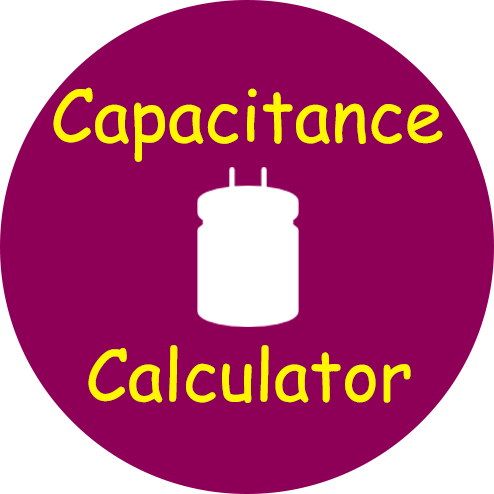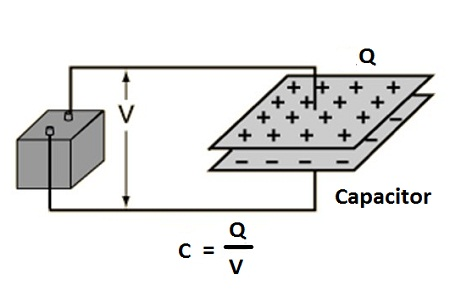# Capacitance Calculator

Use our Capacitance Calculator a free online tool to calculate the capacitance of the electrical circuit based on its charge Q and its voltage V. Enter the values of electrical charge and potential difference to calculate capacitance.

## Capacitance Formula

Capacitance C = Charge Q / Potential Differnece V
C = Q / V### Capacitor

Capacitor is an arrangement of two conductors carrying charges of equal magnitude and opposite sign and separated by an insulating medium.The net charge on the capacitor as a whole is zero. When we say that a capacitor has a charge Q, we mean that positively charged conductor has a charge +Q and the negatively charged conductor has a charge -Q. The positively charged conductor is at a higher potential than negatively charged conductor. The potential difference V between the conductors is proportional to the magnitude of charge Q and the ratio Q/V is known as capacitance C of the capacitor.

The SI unit to measure the capacitance of the capacitor is Farads (F).